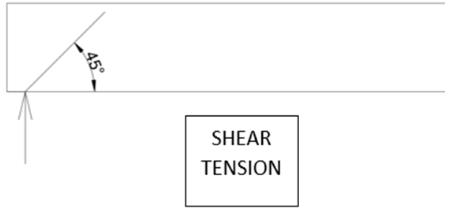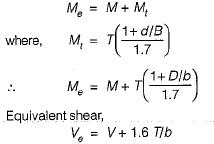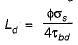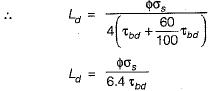Courses

# Test: Bond, Anchorage, Development Length & Torsion

## 10 Questions MCQ Test GATE Civil Engineering (CE) 2022 Mock Test Series | Test: Bond, Anchorage, Development Length & Torsion

Description
This mock test of Test: Bond, Anchorage, Development Length & Torsion for Civil Engineering (CE) helps you for every Civil Engineering (CE) entrance exam. This contains 10 Multiple Choice Questions for Civil Engineering (CE) Test: Bond, Anchorage, Development Length & Torsion (mcq) to study with solutions a complete question bank. The solved questions answers in this Test: Bond, Anchorage, Development Length & Torsion quiz give you a good mix of easy questions and tough questions. Civil Engineering (CE) students definitely take this Test: Bond, Anchorage, Development Length & Torsion exercise for a better result in the exam. You can find other Test: Bond, Anchorage, Development Length & Torsion extra questions, long questions & short questions for Civil Engineering (CE) on EduRev as well by searching above.
QUESTION: 1

### The diagonal tension Which results in ultimate failure due to development of diagonal tension cracks, depends on

Solution:

Diagonal tension is caused by shear stress = Shear Force/ (b.d)
Thus, higher the effective depth lower will be the shear stress and lower will be the length of the crack.
Moreover, the shear tension crack develops only in the shear span. Lower the shear span (where shear force is constant) lower will be the length of the crack.QUESTION: 2

### The development length in compression for a 20 mm diameter deformed bar of grade Fe-415 embedded in concrete of grade M-25, whose design bond stress is 1.40 N/mm2 is

Solution:

For bars in compression the value of bond stress is increased by 25%.
For deformed bars value of bond stress is increased by 60%

QUESTION: 3

### The main reason for providing number of reinforcing bars at a support in a simply supported beam is to resist in that zone

Solution:

Reinforcing bars are provided at the support to develop adequate bond between concrete and steel, so that adequate bond stress is developed between them within development length.

QUESTION: 4

Consider the following statements:
1. Development length includes anchorage value of hooks in tension reinforcement.
2. The development length of each bar of bundled bars shall be that for the individual bar, increased by, 33% for three bars in contact.
3. Deformed bars may be used without end anchorage provided development length requirement is satisfied.
Which of these statements is/are correct?

Solution:

In case of bundled bars in contact the development length is increased than that for individual bar by,
1. 10 % for two bars in contact
2. 20% for three bars in contact
3. 33% for four bars in content

QUESTION: 5

An RC structural member rectangular in cross section of width b and depth D is subjected to a combined action of bending moment M and torsional moment T. The longitudinal reinforcement shall be designed for a moment Me given by

Solution:

The longitudinal reinforcement shall be designed to resist an equivalent bending moment,QUESTION: 6

Which one of the following is the correct expression to estimate the development length of deformed reinforcing bar as per IS code in limit state design?

where φ is diameter of reinforcing bar, σs is the stress in the bar at a section and ζbd is bond stress

Solution:

As per clause 26.2.1 of IS:456-2000, the development length Ld is given by,where,

φ = nominal diameter of bar
σs = stress in the bar at the section considered at design load
ζbd = design bond stress for plain bars

The value of ζbd should be increased by 60% for deformed bars.QUESTION: 7

When HYSD bars are used in place of mild steel bars in a beam, the bond strength

Solution:

When HYSD bars are used in place of mild steel bars bond stress is increased by 60%.

QUESTION: 8

What is the bond stress acting parallel to the reinforcement on the interface between bar and concrete?

Solution:
QUESTION: 9

What is the anchorage value of a standard hook of a reinforcement bar of diameter D?

Solution:

As per clause 26.2.2.1 of IS : 456-2000, the anchorage value of a standard U-type hook shall be equal to 16 times the diameter of the bar

QUESTION: 10

The bond strength between steel and concrete is due to

Solution: